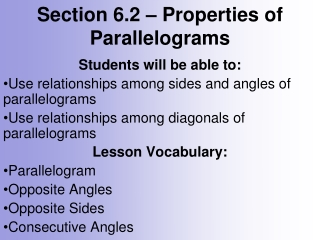DownloadDownload PresentationSection 6.2 – Properties of Parallelograms

# Section 6.2 – Properties of Parallelograms

Download Presentation## Section 6.2 – Properties of Parallelograms

- - - - - - - - - - - - - - - - - - - - - - - - - - - E N D - - - - - - - - - - - - - - - - - - - - - - - - - - -
##### Presentation Transcript

1. Section 6.2 – Properties of Parallelograms Students will be able to: Use relationships among sides and angles of parallelograms Use relationships among diagonals of parallelograms Lesson Vocabulary: Parallelogram Opposite Angles Opposite Sides Consecutive Angles

2. A parallelogram is a quadrilateral with both pairs of opposite sides parallel. Essential Understanding: Parallelograms have special properties regarding their sides, angles, and diagonals.

3. In a quadrilateral, opposite sides do not share a vertex and opposite angles do not share a side.

4. Practice Problem 1: Find the value(s) of the variable(s) in each parallelogram.

5. Angles of a polygon that share a side are consecutive angles. A Parallelogram has opposite sides that are parallel and its consecutive angels are same side interior angles.

6. Problem 1b: Find the value of x in each parallelogram.

7. Problem 1: What is <P in Parallelogram PQRS?

8. Reminder: Solving Systems of Equations: Using Substitution: Using Elimination: Solve one equation for a variable Multiply (if necessary) to get 1 variable Substitute that value in the second to be opposites equation for the given variable. Add or Subtract Equations from Solve for one variable each other Solve for one variable Sub that value into either equation to Sub that value into either equation to get get value of other variable. value of other variable.

9. Problem 1: Solve a system of linear equations to find the values of x and y in Parallelogram KLMN. What are KM and LN?

10. Problem 2: Solve a system of linear equations to find the values of x and y in Parallelogram PQRS. What are PR and SQ?

11. Extra Problems: Find the measures of the numbered angles for each parallelogram.